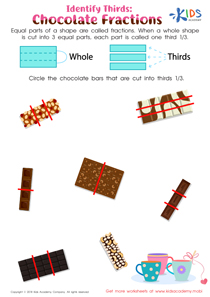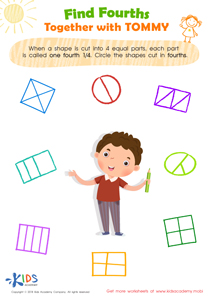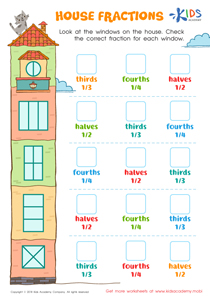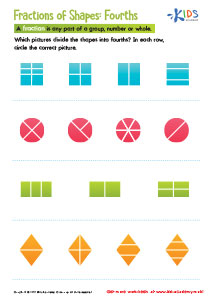# Math Lesson - Cut Rectangles: Thirds and Fourths, Grade 2

• ### Activity 1 / Identify Thirds: Chocolate Fractions WorksheetWhen a shape is divided into more than one equal part, these parts are called fractions. By now, your little ones probably already know that two parts are called a half (½).  But, when a whole shape is divided into three equal parts, each part is called one third (1/3). Show your child this worksheet full of pictures of chocolate bars cut in three equal parts. Then, ask them to circle the pictures with chocolate bars cut into one thirds.

• ### Activity 2 / Find Fourths Together with Tommy WorksheetTeaching your children about fractions can be stressful, not only for you, but for your children as well. Make their difficulties easier with this colorful worksheet. By now, your child should know that dividing a whole shape in two equal parts is called a half, and three equal parts is called a one third. Now, teach them about dividing into four parts, which is called a one fourth (¼). Ask them to circle the shapes in this pdf that are cut in one fourths.

• ### Activity 3 / House Fractions WorksheetAs your child learns more and more about wholes, fractions, halves, one thirds, and one fourths, you might want to test their knowledge a little bit. Use this worksheet to see just how much of the exercises your youngster was able to understand. In the exercise, your child is to look at the windows of the house. Then, check the correct fraction that the window is divided in from the options provided.

• ### Activity 4 / Fractions of Shapes WorksheetSometimes kids have a hard time learning fractions, but when kids are presented with fractions in a variety of ways, the concept is easily understood. This fractions of shapes printable worksheet will allow your child to understand fractions by seeing how fractions apply to familiar geometric shapes.